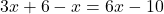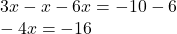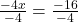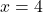## Modeling Equations 3x + 6 – X= 6x – 10 A x= -4 B x = 3 C X=-3 D x = 4​

Question

Modeling Equations 3x + 6 – X= 6x – 10 A
x= -4 B x = 3 C X=-3 D x = 4​

in progress 0
2 weeks 2021-08-28T18:43:03+00:00 1 Answers 0 views 0

1. ## D. x = 4

Step-by-step explanation:Collect like terms and simplifyDivide both sides of the equation by 4Simplify